### Home > CALC > Chapter 4 > Lesson 4.2.4 > Problem4-88

4-88.
1. Rewrite the following integral expressions as single integrals. Homework Help ✎

1.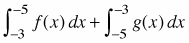2.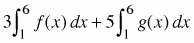3.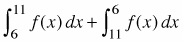4.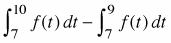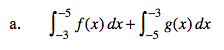$\int_{a}^{b}f(x)dx=\int_{b}^{a}-f(x)dx$

$\int_{-5}^{-3}\left (g(x)-f(x) \right )dx$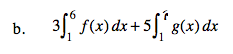Translation: three times the area under f(x) between x = 1 and x = 6 + five times the same area. Write this as a single integral.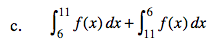See hint (a) and then see hint (c).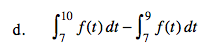Since an integral is the area underneath or above a curve, if you take a smaller portion of that area and subtract it from a larger portion, both starting at the same point, what are you left with?